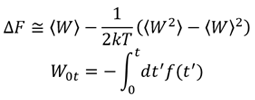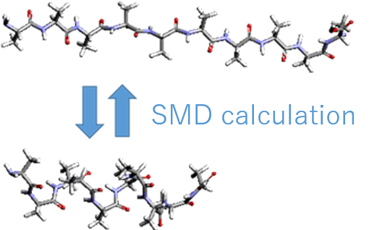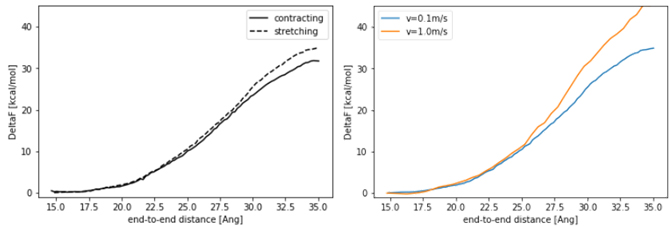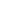Full Atomistic MD
Molecular structure / Affinity / Solubility
Life Science

# Free energy change of polypeptides by Steered MD

Analysis of the free energy change along the reaction coordinate

## Objectives and Methods

In Steered Molecular Dynamics (SMD), the structure of a system is forced to change by virtual particles, and the work done on the system, W, can be evaluated from the force, f, applied to the virtual particles, and the free energy change due to the structural change, ΔF, can be calculated.Using this method, we calculated the free energy change during the conformational change from the elongated state to the helix state using the end-to-end distance of the polypeptide as the reaction coordinate. As shown in Fig. 1, we modeled a decamer of alanine as a polypeptide and set up a GAFF force field; the N-terminus was fixed, a virtual particle was bound to the C-terminus, and SMD calculations were performed to change the end-to-end distance. The ensemble average of work W was calculated from 10 trajectories. The velocity v of the virtual particle was 0.1 m/s and 1.0 m/s.Fig. 1 Model used in the calculation (decamer of alanine). The N-terminus is fixed and the C-terminus is moved.

## Analysis Results

Figure 2 shows the calculated free energy change plotted against the distance between the ends.

On the left side of the figure are the free energy changes calculated with v=0.1 m/s, for the contracting process from the elongated state (contracting) and the stretching process from the helix (stretching).

The results are almost the same for both processes, although there is a discrepancy in the region where the distance between the ends is long. Ideally, the same free energy difference should be obtained regardless of the process.

On the right side of the figure, we compare the free energy change of the elongation process with respect to the velocity of the virtual particle, and the free energy change is smaller when v=0.1m/s is used.

The calculated free energy difference is larger than the result in reference , which is mainly due to the difference in the force field.Figure 2 (Left) Free energy change of the elongated-helix state of a polypeptide. (Right) Free energy change for different virtual particle velocities.

• Reference
•  S. Park, et al., “Free Energy Calculation from Steered Molecular Dynamics Simulations Using Jarzynski ’ s Equality,” J. Chem. Phys., 119, 3553, (2003).This website uses cookies to improve functionality and performance. If you continue browsing the site, you are giving implied consent to the use of cookies on this website. If you want to know more or refuse consent, read our Cookie Policy.

Accept